# Analysis of variance: ANOVA (1 way)

Analysis of variance is the technique to use when you might otherwise be considering a large number of pairwise F and t tests, i.e. where you want to know whether a factor with more than 2 levels is a useful predictor of a dependent variable.

For example, cuckoo_eggs.csv contains data on the length of cuckoo eggs laid into different host species, the (meadow) pipit, the (reed) warbler, and the wren.

A box-and-whisker plot is a useful way to view data of this sort:

```cuckoo.eggs<-read.csv( "H:/R/cuckoo_eggs.csv" )
boxplot(
egg.length ~ species,
data = cuckoo.eggs,
xlab = "Host species",
ylab = "Egg length / mm"
)```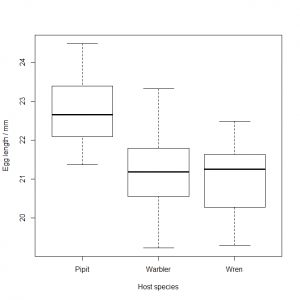You might be tempted to try t testing each pairwise comparison (pipit vs. wren, warbler vs. pipit, and warbler vs. wren), but a one-factor analysis of variance (ANOVA) is what you actually want here. ANOVA works by fitting individual means to the three levels (warbler, pipit, wren) of the factor (host species) and seeing whether this results in a significantly smaller residual variance than fitting a simple overall mean to the entire data set.

Conceptually, this is very similar to what we did with linear regression: ANOVA compares the residuals on the model represented by the “y is a constant” graph below: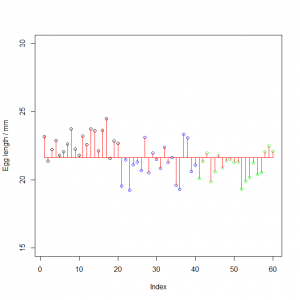…with a model where three individual means have been fitted, the “y varies by group” model: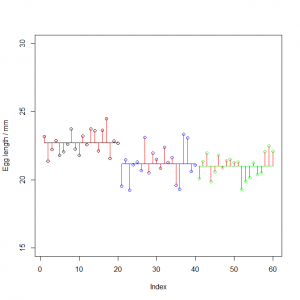It’s not immediately obvious that fitting three separate means has bought us much: the model is more complicated, but the length of the red lines doesn’t seem to have changed hugely. However, R can tell us precisely whether or not this is the case. The syntax for categorical model fitting is `aov()`, for analysis of variance:

`aov( egg.length ~ species, data=cuckoo.eggs )`
```Call:
aov(formula = egg.length ~ species, data = cuckoo.eggs)
Terms:
species Residuals
Sum of Squares  35.57612  55.85047
Deg. of Freedom        2        57
Residual standard error: 0.989865
Estimated effects may be unbalanced```

As with linear regression, you may well wish to save the model for later use. It is traditional to display the results of an ANOVA in tabular format, which can be produced using `anova()`

```cuckoo.model<-aov( egg.length ~ species, data=cuckoo.eggs )
anova( cuckoo.model )```
```Analysis of Variance Table
Response: egg.length
Df Sum Sq Mean Sq F value    Pr(>F)
species    2 35.576 17.7881  18.154 7.938e-07 ***
Residuals 57 55.850  0.9798
---
Signif. codes:  0 ‘***’ 0.001 ‘**’ 0.01 ‘*’ 0.05 ‘.’ 0.1 ‘ ’ 1```

The “y is a constant” model has only one variance associated with it: the total sum of squares of the deviances from the overall mean (SST, or SSY, whichever you prefer) divided by the degrees of freedom in the data set (n−1).

The “y varies by group” model decomposes this overall variance into two components.

• The first component (SSA) is associated with categorising the data into k groups (k=3 here). It is the sum of squares of the difference between the group-specific mean of each data point from the overall mean, divided by the number of degrees of freedom those k means have, (k−1). This represents the between group variation in the data.
• The second component (SSE) is the residual variance, left unexplained by assigning k different means to each of the k groups. It is the sum of squares of the data points from their group-specific mean, divided by the degrees of freedom left after estimating those k individual means, (nk). This represents the within group variation in the data.

In the ANOVA table, the `Sum Sq` is the sum of the squares of the deviances of the data points from a mean. The `Df` is the degrees of freedom, and the `Mean Sq` is the sum of squares divided by the degrees of freedom, which is the corresponding variance.

The `F value` is the mean-square for `species` divided by the mean-square of the `Residuals`. The p value indicates that categorising the data into three groups does make a significant difference to explaining the variance in the data, i.e. estimating three separate mean for each host species, rather than one grand mean, does make a significant difference to how well we can explain the data. The length of the eggs the cuckoo lays does vary by species.

Compare this with linear regression, where you’re trying to find out whether y=a+bx is a better model of the data than y=. This is very similar to ANOVA, where we are trying to find out whether “y varies by group, i.e. the levels of a factor” is a better model than “y is a constant, i.e. the overall mean”.

You might well now ask “but which means are different?” This can be investigated using `TukeyHSD()` (honest significant differences) which performs a (better!) version of the pairwise t test you were probably considering at the top of this post.

`TukeyHSD( cuckoo.model )`
```  Tukey multiple comparisons of means
95% family-wise confidence level
Fit: aov(formula = egg.length ~ species, data = cuckoo.eggs)
\$species
Warbler-Pipit -1.5395 -2.2927638 -0.7862362 0.000023
Wren-Pipit    -1.7135 -2.4667638 -0.9602362 0.000003
Wren-Warbler  -0.1740 -0.9272638  0.5792638 0.843888```

This confirms that – as the box-and-whisker plots suggested – the eggs laid in wren and warbler nests are not significantly different in size, but those laid in pipit nests are significantly larger than those in the warbler or wren nests.

ANOVA has the same sorts of assumption as the F and t tests and as linear regression: normality of residuals, homoscedacity, representativeness of the sample, no error in the treatment variable, and independence of data points. You should therefore use the same checks after model fitting as you used for linear regression:

`plot( residuals( cuckoo.model ) ~ fitted( cuckoo.model ) )`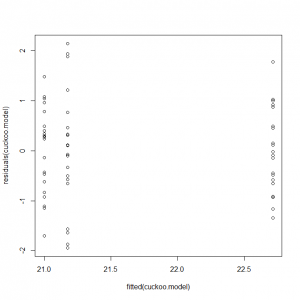We do not expect a starry sky in the residual plot, as the fitted data are in three discrete levels. However, if the residuals are homoscedastic, we expect them to be of similar spread in all three treatments, i.e. the residuals shouldn’t be more scattered around the zero line in the pipits than in the wrens, for example. This plot seems consistent with that.

`qqnorm( residuals( cuckoo.model ) )`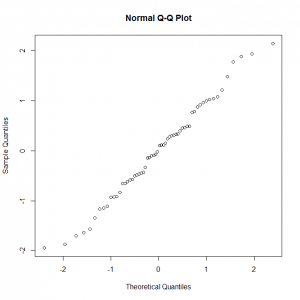On the normal Q-Q plot, we do expect a straight line, which – again – we appear to have (although it’s a bit jagged, and a tiny bit S-shaped). We can accept that the residuals are more-or-less normal, and therefore that the analysis of variance was valid.

# Exercises

Analyse the following data set with ANOVA

1. The file venus_flytrap.csv contains data on the wet biomass (g) of Venus flytraps prevented from catching flies (control), allowed to catch flies, or prevented from catching flies but instead given fertiliser applied to the soil. Does the feeding treatment significantly affect the biomass? If so, which of the three means differ significantly, and in what directions? Have a look at the residuals in the same way as you did for the regression. How do they look?

1. Venus flytrap biomass data
```venus.flytrap<-read.csv("H:/R/venus_flytrap.csv")
plot(
biomass ~ feed,
data = venus.flytrap,
xlab = "Feeding treatment",
ylab = "Wet biomass / g",
main = expression("Venus flytrap feeding regime affects wet biomass")
)```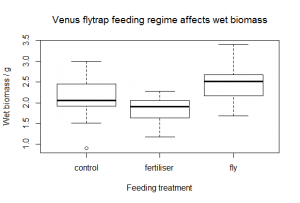```flytrap.model<-aov( biomass ~ feed, data = venus.flytrap )
anova( flytrap.model )```
```Analysis of Variance Table
Response: biomass
Df  Sum Sq Mean Sq F value    Pr(>F)
feed      2  5.8597 2.92983  22.371 1.449e-08 ***
Residuals 87 11.3939 0.13096
---
Signif. codes:
0 '***' 0.001 '**' 0.01 '*' 0.05 '.' 0.1 ' ' 1```
`TukeyHSD( flytrap.model )`
```  Tukey multiple comparisons of means
95% family-wise confidence level
Fit: aov(formula = biomass ~ feed, data = venus.flytrap)
\$feed
fertiliser-control -0.3086667 -0.53147173 -0.0858616 0.0039294
fly-control         0.3163333  0.09352827  0.5391384 0.0030387
fly-fertiliser      0.6250000  0.40219493  0.8478051 0.0000000```

The feeding treatment makes a significant different to the wet biomass (F=22.3, p=1.45 × 10−8) and a Tukey HSD test shows that all three means are different, with the fly treatment having a beneficial effect (on average, the fly-treated plants are 0.32 g heavier than the control plants), but the fertiliser has an actively negative effect on growth, with these plants on average being 0.31 g lighter than the control plants.

To plot the residuals, we use the same code as for the linear regression:

`plot( residuals(flytrap.model) ~ fitted(flytrap.model) )`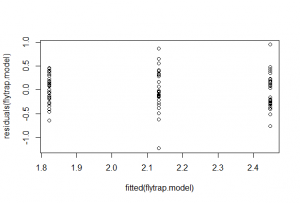There is perhaps a bit more scatter in the residuals of the control (the ones in the middle), but nothing much to worry about.

`qqnorm( residuals( flytrap.model ) )`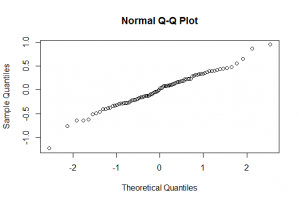On the normal Q-Q plot, we do expect a straight line, which – again – we appear to have. We can accept that the residuals are essentially homoscedastic and normal, and therefore that the analysis of variance was valid.

Next up… Two-way ANOVA.

•• student on 2017-07-25 at 14:51

Hi, I don’t quite understand how you reached this conclusion from the tukeyHSD
“This confirms that – as the box-and-whisker plots suggested – the eggs laid in pipit and warbler nests are not significantly different in size, but those laid in wren nests are significantly smaller than those in the warbler or pipit nests”

the p value 0.05 for wren-warbler.

•• polypompholyx on 2017-07-28 at 11:33
Author

Yeah, that was a complete mess: sorry! I’ve corrected it now:

“This confirms that – as the box-and-whisker plots suggested – the eggs laid in wren and warbler nests are not significantly different in size, but those laid in pipit nests are significantly larger than those in the warbler or wren nests.”

This site uses Akismet to reduce spam. Learn how your comment data is processed.